Geometric Progression(GP): Formulae based

Chapter 8 Class 11 Sequences and Series
Concept wise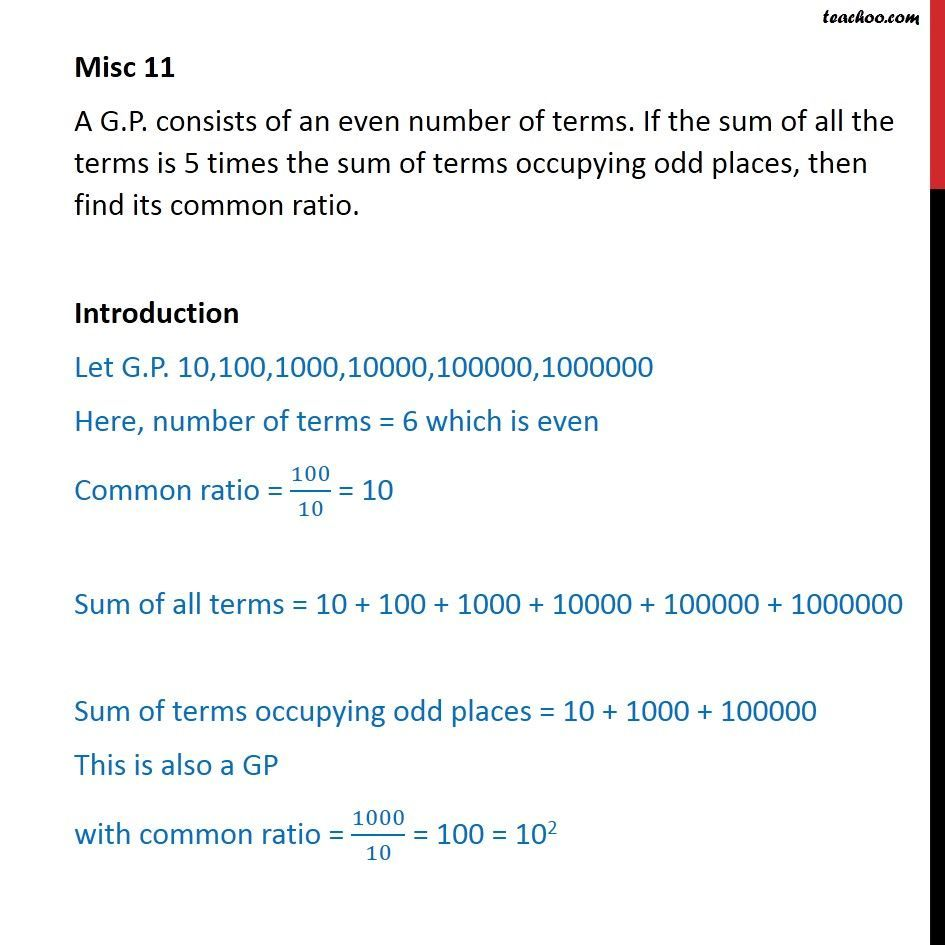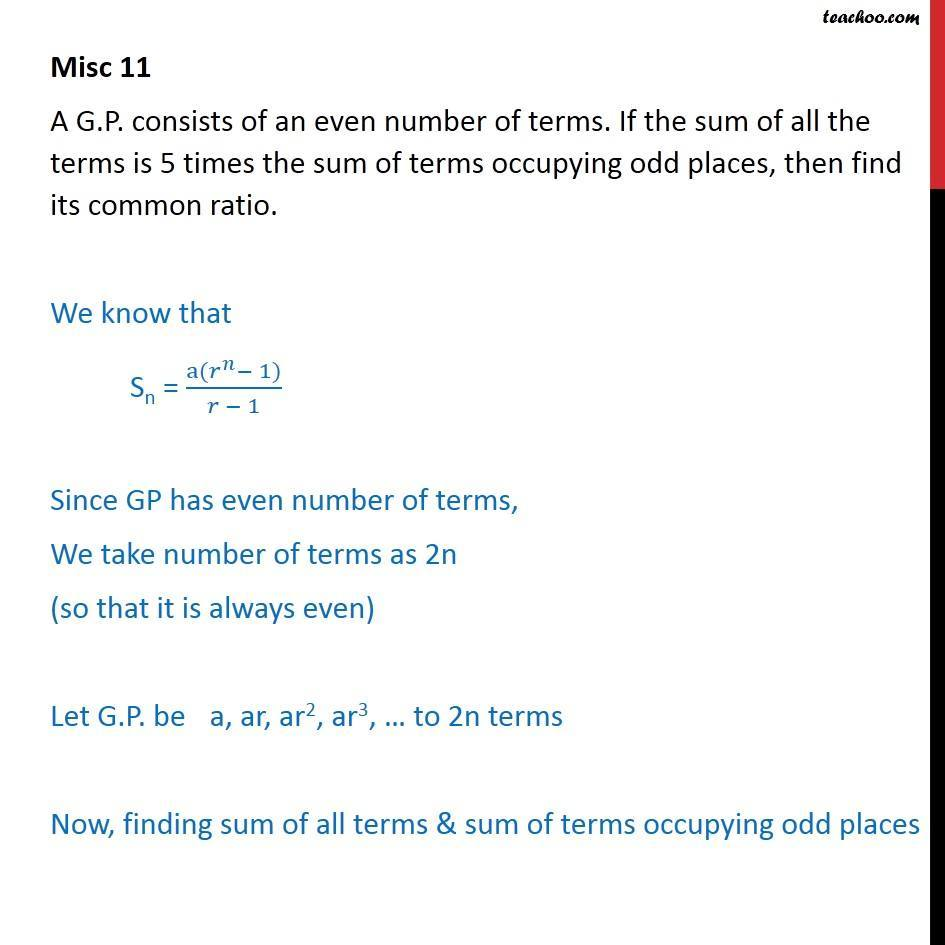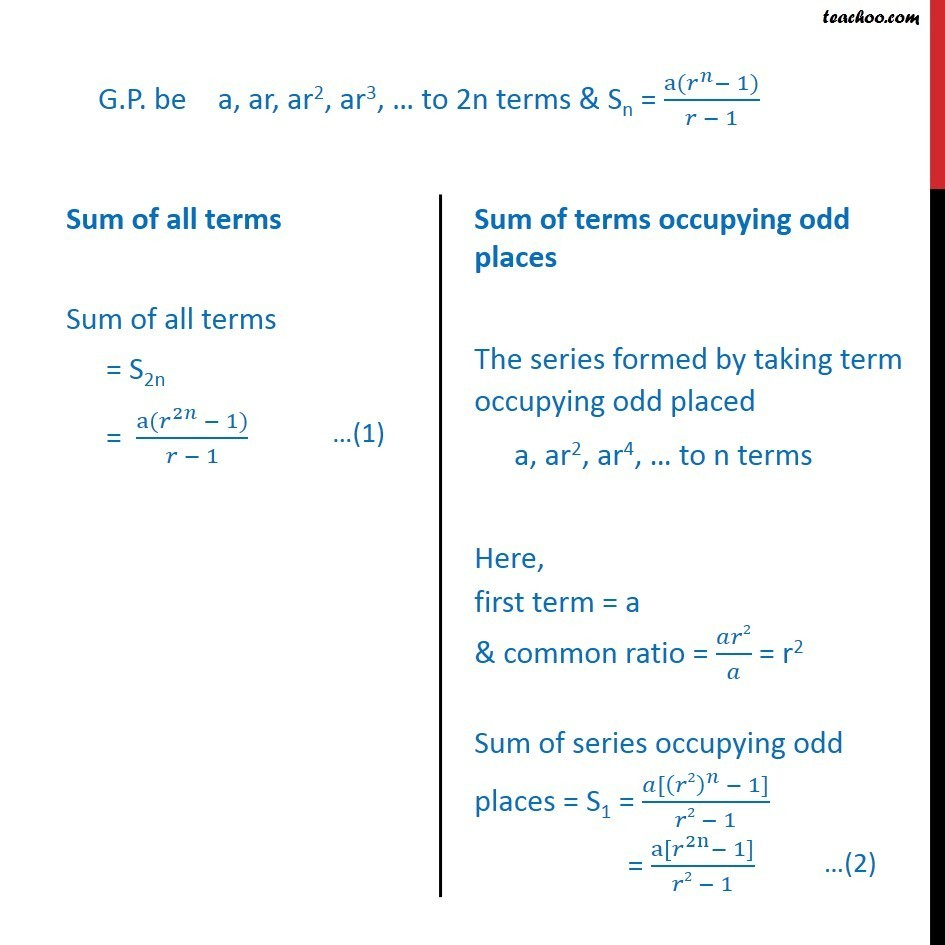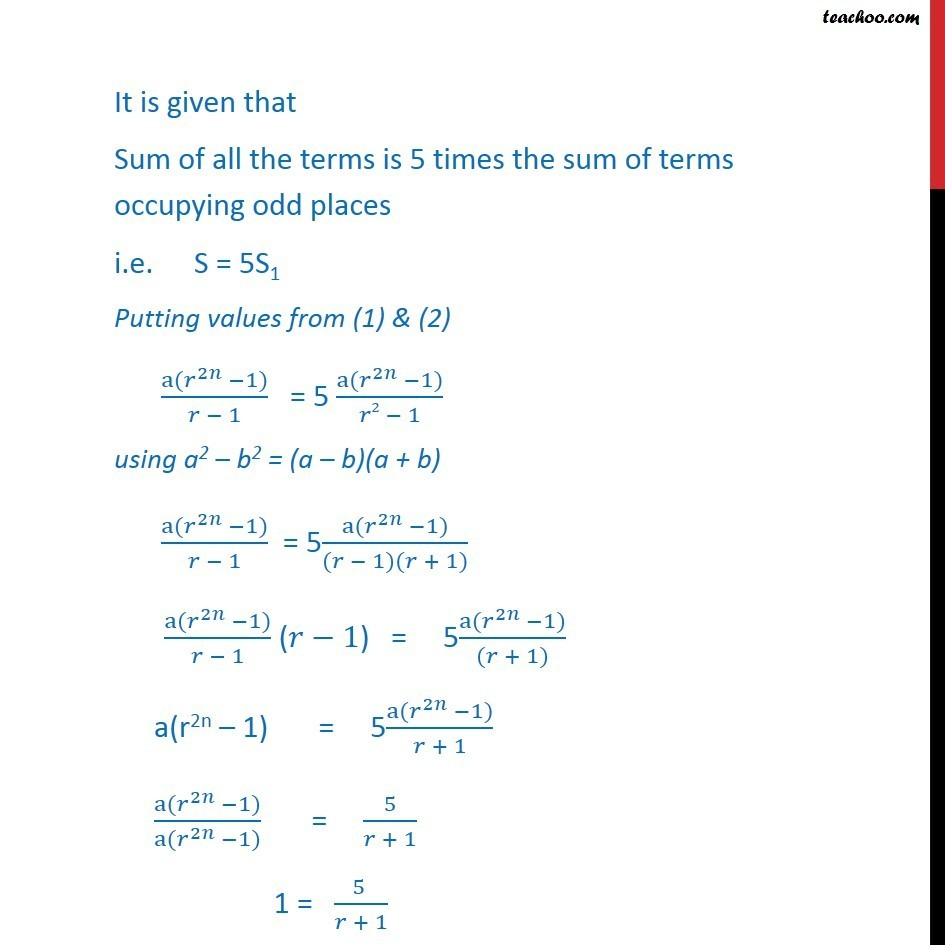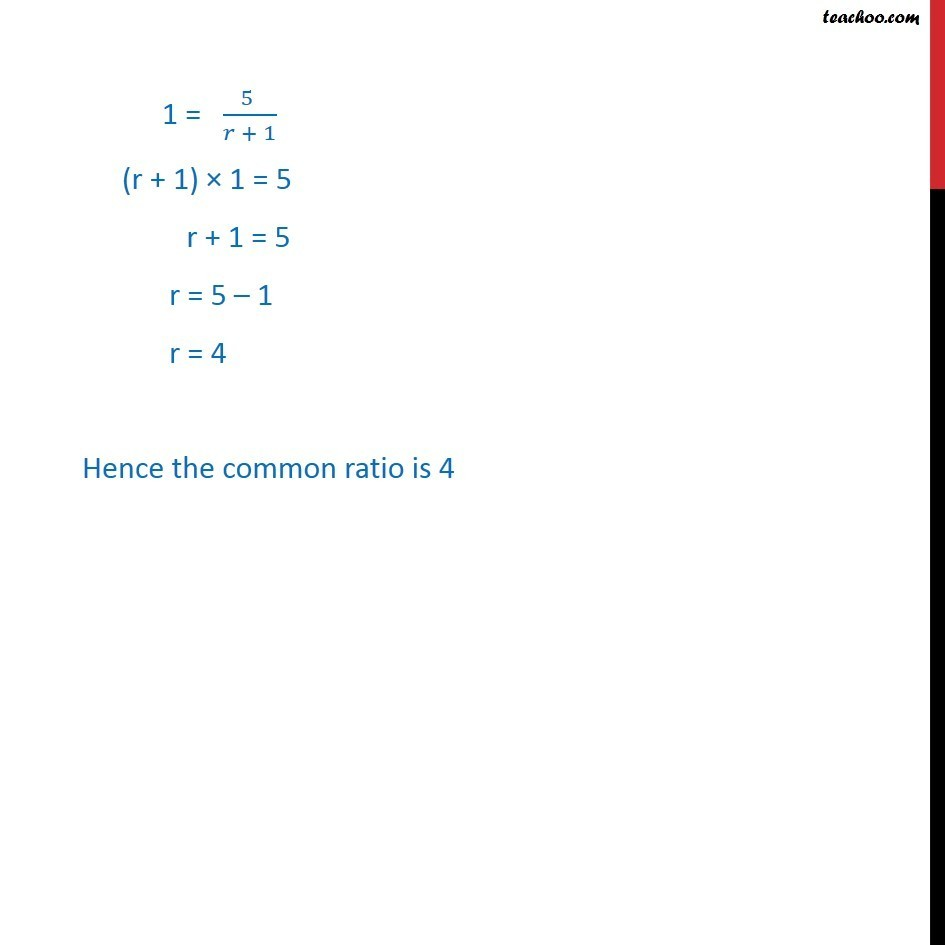Learn in your speed, with individual attention - Teachoo Maths 1-on-1 Class

### Transcript

Misc 5 A G.P. consists of an even number of terms. If the sum of all the terms is 5 times the sum of terms occupying odd places, then find its common ratio. Introduction Let G.P. 10,100,1000,10000,100000,1000000 Here, number of terms = 6 which is even Common ratio = 100/10 = 10 Sum of all terms = 10 + 100 + 1000 + 10000 + 100000 + 1000000 Sum of terms occupying odd places = 10 + 1000 + 100000 This is also a GP with common ratio = 1000/10 = 100 = 102 Misc 5 A G.P. consists of an even number of terms. If the sum of all the terms is 5 times the sum of terms occupying odd places, then find its common ratio. We know that Sn = (a(𝑟^𝑛− 1))/(𝑟 − 1) Since GP has even number of terms, We take number of terms as 2n (so that it is always even) Let G.P. be a, ar, ar2, ar3, … to 2n terms Now, finding sum of all terms & sum of terms occupying odd places G.P. be a, ar, ar2, ar3, … to 2n terms & Sn = (a(𝑟^𝑛− 1))/(𝑟 − 1) It is given that Sum of all the terms is 5 times the sum of terms occupying odd places i.e. S = 5S1 Putting values from (1) & (2) (a(𝑟^2𝑛 −1))/(𝑟 − 1) = 5 (a(𝑟^2𝑛 −1))/(𝑟2 − 1) using a2 – b2 = (a – b)(a + b) (a(𝑟^2𝑛 −1))/(𝑟 − 1) = 5(a(𝑟^2𝑛 −1))/((𝑟 − 1)(𝑟 + 1)) (a(𝑟^2𝑛 −1))/(𝑟 − 1) (𝑟−1) = 5(a(𝑟^2𝑛 −1))/((𝑟 + 1)) a(r2n – 1) = 5(a(𝑟^2𝑛 −1))/(𝑟 + 1) (a(𝑟^2𝑛 −1))/(a(𝑟^2𝑛 −1)) = 5/(𝑟 + 1) 1 = (5 )/(𝑟 + 1) 1 = (5 )/(𝑟 + 1) (r + 1) × 1 = 5 r + 1 = 5 r = 5 – 1 r = 4 Hence the common ratio is 4